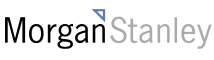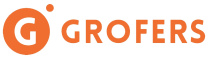New update is available. Click here to update.
Topics

# Vertical Order Traversal

Moderate0/80
Average time to solve is 35m+14 more companies

## Problem statement

Given a binary tree, return the vertical order traversal of the values of the nodes of the given tree.

For each node at position (X, Y), (X-1, Y-1) will be its left child position while (X+1, Y-1) will be the right child position.

Running a vertical line from X = -infinity to X = +infinity, now whenever this vertical line touches some nodes, we need to add those values of the nodes in order starting from top to bottom with the decreasing ‘Y’ coordinates.

Note:
``````If two nodes have the same position, then the value of the node that is added first will be the value that is on the left side.
``````
For example:
``````For the binary tree in the image below.
````````````The vertical order traversal will be {2, 7, 5, 2, 6, 5, 11, 4, 9}.
``````
Detailed explanation ( Input/output format, Notes, Images )
Constraints:
``````1 <= 'T' <= 100
0 <= 'N' <= 3000
0 <= 'VAL' <= 10^5

Where 'VAL' is the value of any binary tree node.

Time Limit: 1 sec
``````
Sample Input 1:
``````2
1 2 3 4 -1 -1 -1 -1 -1
1 -1 2 -1 -1
``````
Sample Output 1:
``````4 2 1 3
1 2
``````
Explanation of Sample Input 1:
``````For the first test case, the vertical order traversal of the given binary tree will be {{4}, {2}, {1}, {3}}.

For the second test case, the vertical order traversal of the given binary tree will be {{1}, {2}}.
``````
Sample Input 2:
``````2
2 1 -1 -1 -1
0 1 2 4 5 3 6 -1 -1 7 -1 -1 -1 -1 -1 -1 -1
``````
Sample Output 2:
``````1 2
4 1 7 0 5 3 2 6
``````
Explanation of Sample Input 2:
``````For the first test case, the vertical order traversal of the given binary tree will be {{1}, {2}}.

For the second test case, the vertical order traversal of the given binary tree will be {{4}, {1, 7}, {0, 5, 3}, {2}, {6}}.
``````Console